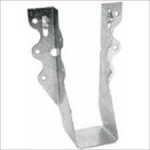# 4×4 or Double 2×4 Bay Roof Purlins?

4×4 or Double 2×4 for 12’ Bay Roof Purlins?

“If you have trusses spaced at 12 feet, can a 4x4x12 or two 2x4x12’s span the distance given the minimal snow loads in Arkansas? I know this is question #2 but what kind of joist hangers do you use (Simpson Number or equivalent) for purlin attachment to trusses?”We typically would use 2×6 #2 on edge for these recessed (between truss pairs) roof purlins. Here are the calculations:

Assumptions:

Roof slope = 4:12 (18.435° roof angle)
Trusses spaced 12-ft. o.c.
Purlin span = 11.75-ft.
Purlin spacing = 24 in.
Purlin size 2″ x 6″ #2
Roof steel dead load = 0.63 psf steel American Building Components catalogue
Roof lumber dead load = 62.4 pcf * 0.55 lbs/ft.3 / (1 + 0.55 lbs/ft.3 * 0.009 * 0.19) * (1 + 0.0019) * 1.5″ / 12 in./ft. * 5.5″ / 12 in./ft. * (12′ – 3″ / 12 in./ft.) / 12′ / (24″ / 12 in./ft.) psf in purlin weight based on 0.55 G NDS = 0.963 psf

Bending Stresses

Fb: allowable bending pressure
Fb‘ = Fb * CD * CM * Ct * CL * CF * Cfu * Ci * Cr
CD = 1.25 NDS 2.3.2
CM: wet service factor
CM = 1 because purlins are protected from moisture by roof
Ct: temperature factor
Ct = 1 NDS 2.3.3
CL: beam stability factor
CL = 1 NDS 4.4.1
CF: size factor
CF = 1 (not applicable to SYP)
Cfu: flat use factor
Cfu = 1 NDS Supplement table 4A
Ci: incising factor
Ci = 1 NDS 4.3.8
Cr: repetitive member factor
Cr = 1.15 NDS 4.3.9
Fb =1000 psi NDS Supplement Table 4-A
Fb‘ = 1000 psi * 1.25 * 1 * 1 * 1 * 1 * 1 * 1 * 1.15
Fb‘ = 1437.5 psi

fb = (purlin_dead_load + Lr) * spacing / 12 * cos(θ) / 12 * (sf * 12 – 3)2 / 8 * 6 / b / d2 * cos(θ)
Lr = 20 psf using the appropriate load calculated above
fb = 21.593 psf * 24″ / 12 in./ft. * cos(18.435) / 12 in./ft. * (12′ * 12 in./ft. – 3″)2 / 8 * 6 / 1.5″ / 5.5″2 * cos(18.435)
fb = 1060 psi ≤ 1437.5 psi; stressed to 73.7 %

Deflection

Δallow: allowable deflection
Δallow = l / 180 IBC table 1604.3
l = 141″
Δallow = 141″ / 180
Δallow = 0.783″
Δmax: maximum deflection
Δmax = 5 * Lr * spacing * cos(θ * π / 180) * (sf * 12 – 3)4 / 384 / E / I from http://www.awc.org/pdf/DA6-BeamFormulas.pdf p.4
E: Modulus of Elasticity
E = 1400000 psi NDS Supplement
I: moment of inertia
I = b * d3 / 12
I = 1.5″ * 5.5″3 / 12
I = 20.796875 in.4
Δmax = 5 * 20 psf / 144 psi/psf * 24″ * cos(18.435° * 3.14159 / 180) * (12′ * 12 in./ft. – 3″)4 / 384 / 1400000 psi / 20.796875 in.4
Δmax = 0.559″ ≤ 0.783″

2×4 #2 and 4×4 #2 Southern Pine have Fb values of 1100

Sm (Section Modulus) of a 2×6 is 7.5625; (2) 2×4 nailed together would be 1.5″ width x 3.5″ depth^2 x 2 members = 6.125 I would = 10.71875; 4×4 would be 7.146 with I = 12.5052

The (2) 2×4 would be stressed to 82.7% in bending however Δmax = 1.085″ so would fail due to being over deflection limits

How about a 4×4? 70.9% in bending Δmax = 0.9296″ so would also fail due to being over deflection limits

For our 2×6 purlins, we specify a Simpson LU26